# Fluid Classification

Fluid classification is the somewhat ambiguous task of labeling a reservoir fluid from a range of categories like volitile oil, gas condensate or dry gas. This task is essentially the same as classifying a reservoir type. There are several potential metrics used to classify a fluid, and in this article the solution gas-oil ratio (GOR) and phase envelope will be used to do fluid classification.

To show some of the ambiguity in classifying a reservoir fluid, two different definitions of different fluid classes are given below for the solution GOR.

Reservoir Type GOR (scf/STB)
Black Oil <1000
Volatile Oil 1000-3000
Near-Critical ~3000
Gas Condensate 3000-30000
Wet Gas 30000-100000
Dry Gas $$\infty$$

Excerpt 1: Figure taken from the SPE Monograph Phase Behavior1 page 12 showing range of GOR and OGR for different types of fields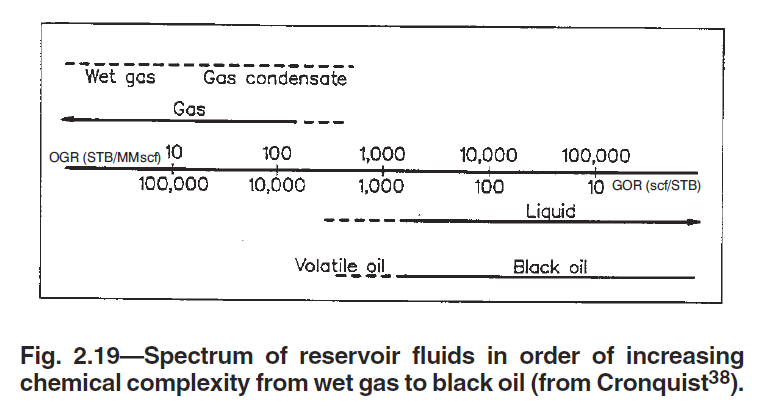In the following sections, another common definition (with somewhat less ambiguity) is given by associating a reservoir fluid class with its relationship to the reservoir fluid's critical temperature.

## Black-Oil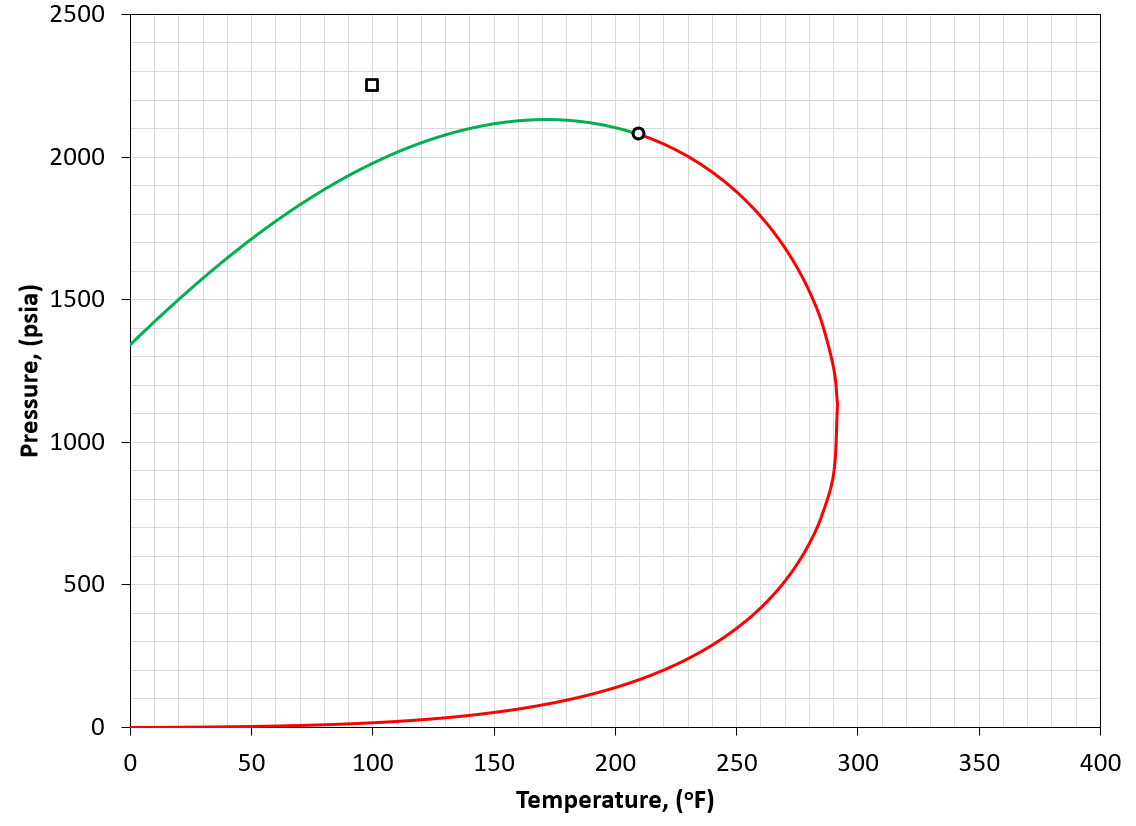Figure 1: An example of a PT phase envelope (bubble point in green and dew point in red) for a black-oil reservoir. The reservoir condition is marked by a black square marker.

A black-oil fluid is defined by having a reservoir temperature much less than the critical temperature of the reservoir fluid (i.e. $$T_{R}<<T_{c}$$). An example is given in Figure 1. For the black-oil classification, a GOR of less than 1000 scf/STB is also a good indication as the definition of "much less" is ambiguous.

## Volatile Oil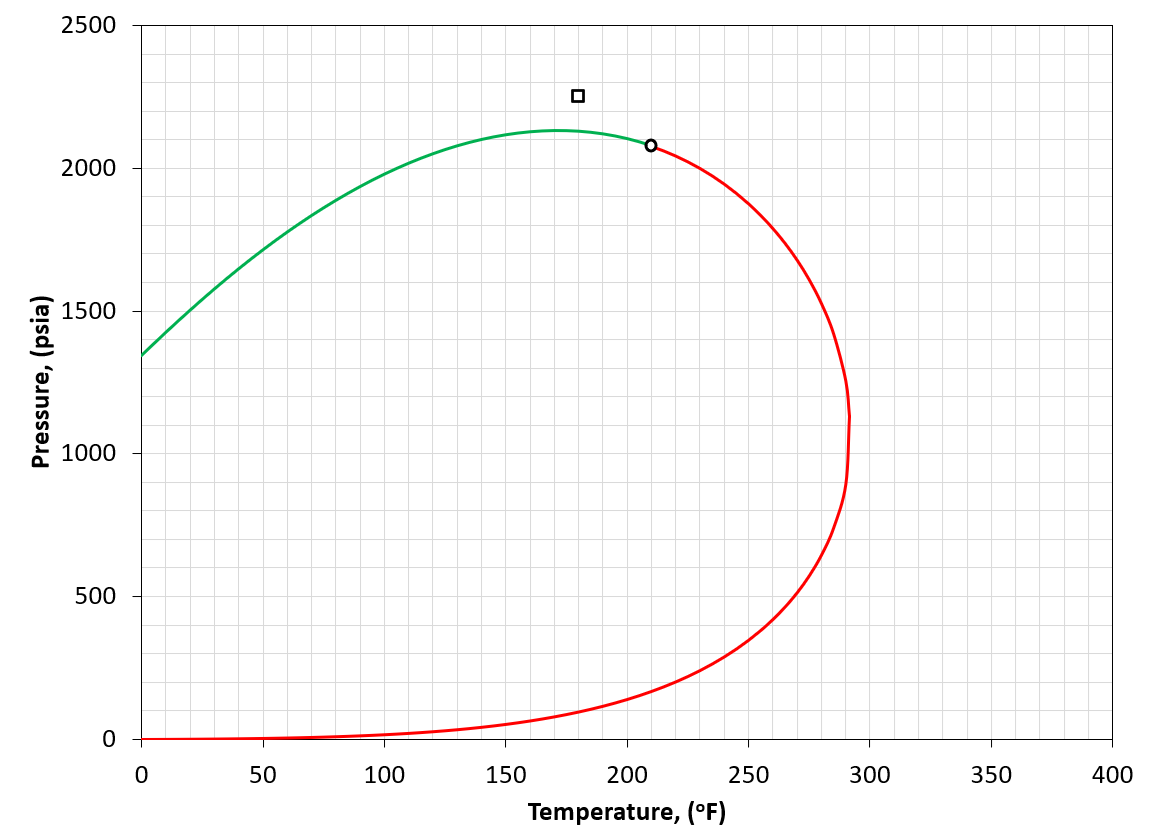Figure 2: An example of a PT phase envelope (bubble point in green and dew point in red) for a volatile oil reservoir. The reservoir condition is marked by a black square marker.

A volatile oil fluid is defined by having a reservoir temperature somewhat less than the critical temperature of the reservoir fluid (i.e. $$T_{R}<T_{c}$$). An example is given in Figure 2. For the volatile oil classification, a GOR of 1000 to 3000 scf/STB is also a good indication as the definition of "somewhat less" is ambiguous.

## Near-Critical Fluid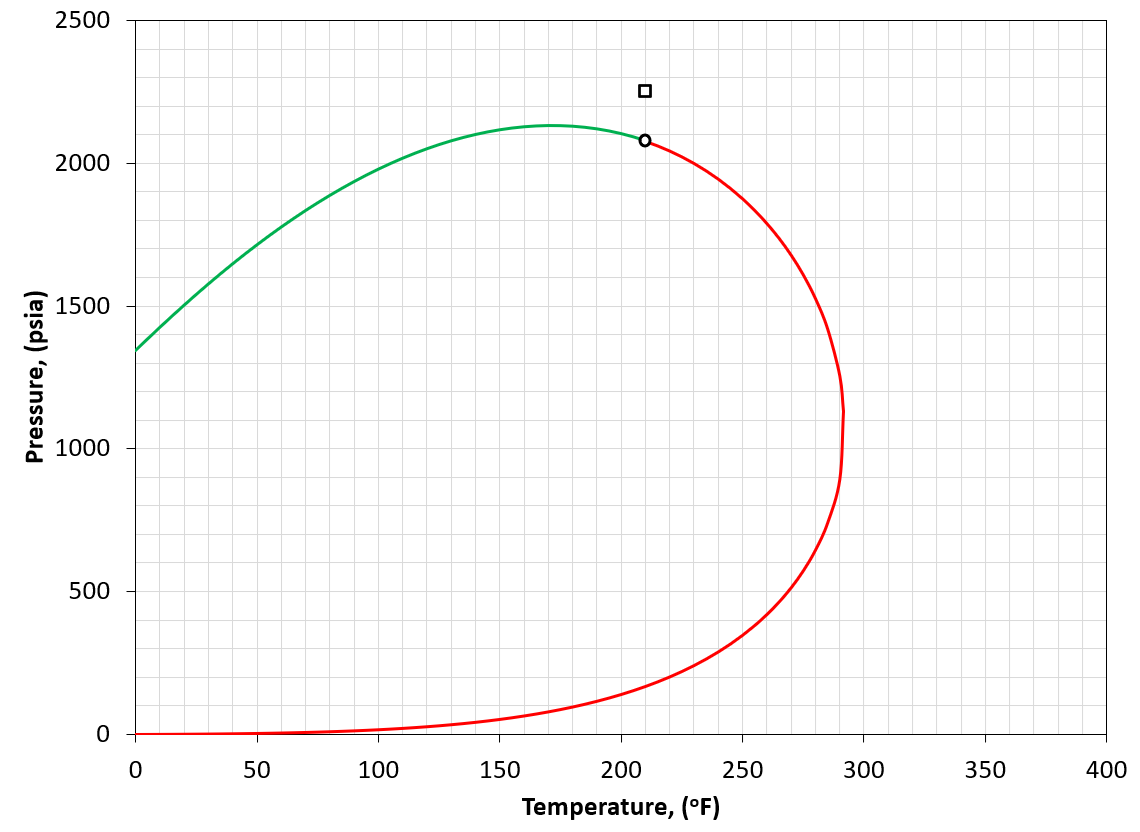Figure 3: An example of a PT phase envelope (bubble point in green and dew point in red) for a near-critical oil reservoir. The reservoir condition is marked by a black square marker.

A near critical fluid is defined by having a reservoir temperature close to the critical temperature of the reservoir fluid (i.e. $$T_{R} \approx T_{c}$$). An example is given in Figure 3. For the near critical fluid classification, a GOR of around 3000 scf/STB is also a good indication as the definition of "close" is ambiguous.

## Gas Condensate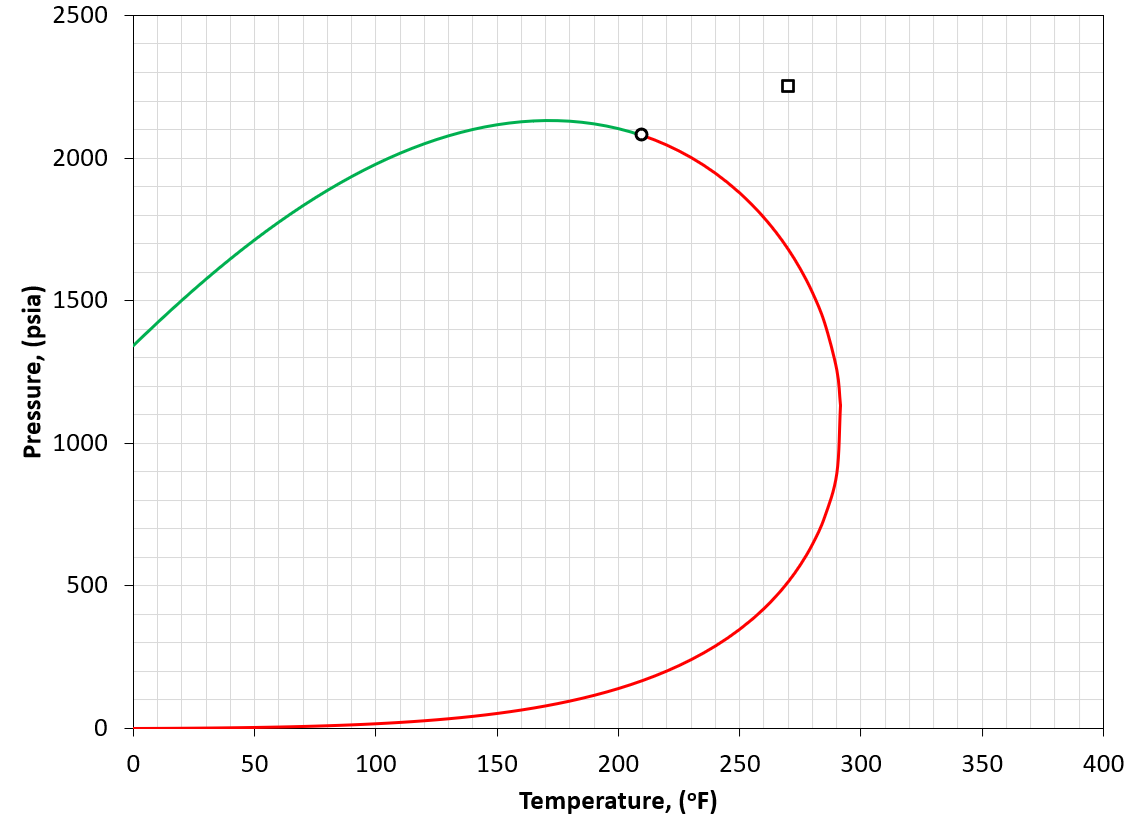Figure 4: An example of a PT phase envelope (bubble point in green and dew point in red) for a gas condensate reservoir. The reservoir condition is marked by a black square marker.

A gas condensate is defined by having a reservoir temperature between the critical temperature and the cricondentherm of the reservoir fluid (i.e. $$T_{c}<T_{R}<T_{cric}$$). An example is given in Figure 4. The general idea of this classification is that if we reduce the pressure, the original gas-like fluid will condense retrograde fluid.

## Wet Gas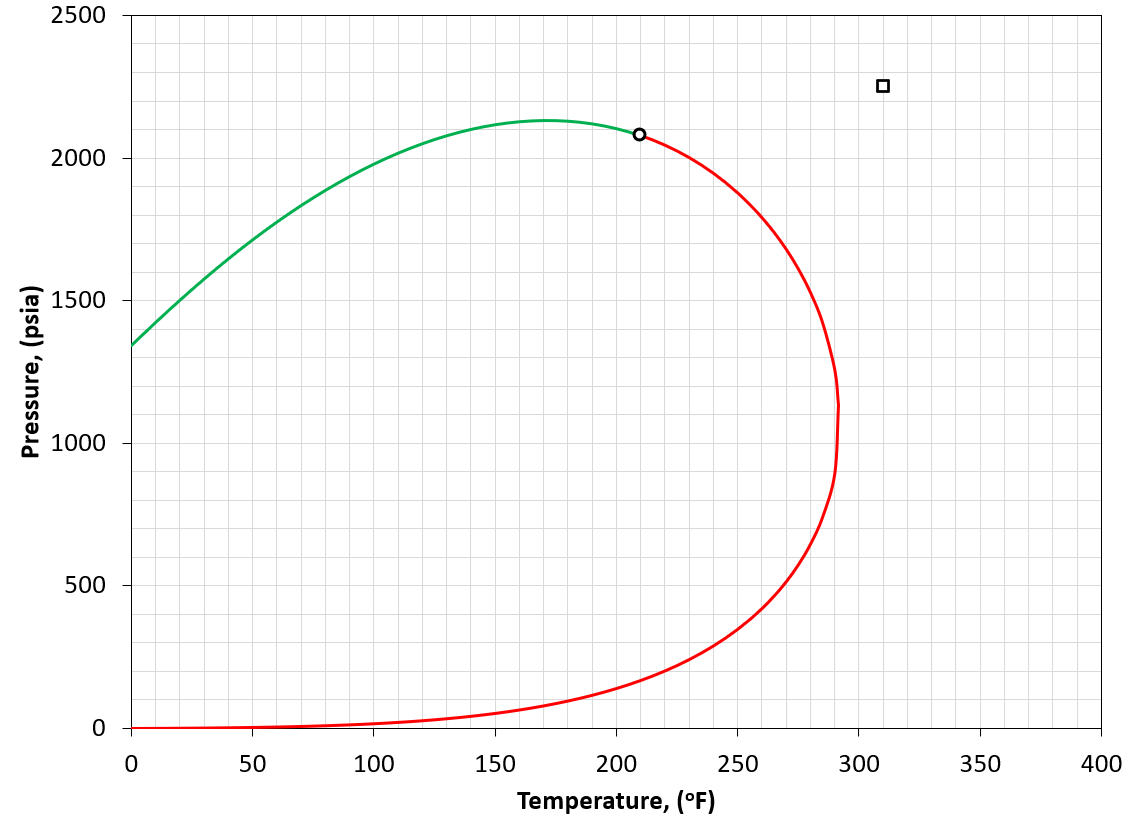Figure 5: An example of a PT phase envelope (bubble point in green and dew point in red) for a wet gas reservoir. The reservoir condition is marked by a black square marker.

A wet gas is defined by having a reservoir temperature somewhat more than the cricondentherm of the reservoir fluid (i.e. $$T_{R}>T_{cric}$$). An example is given in Figure 5.

## Dry Gas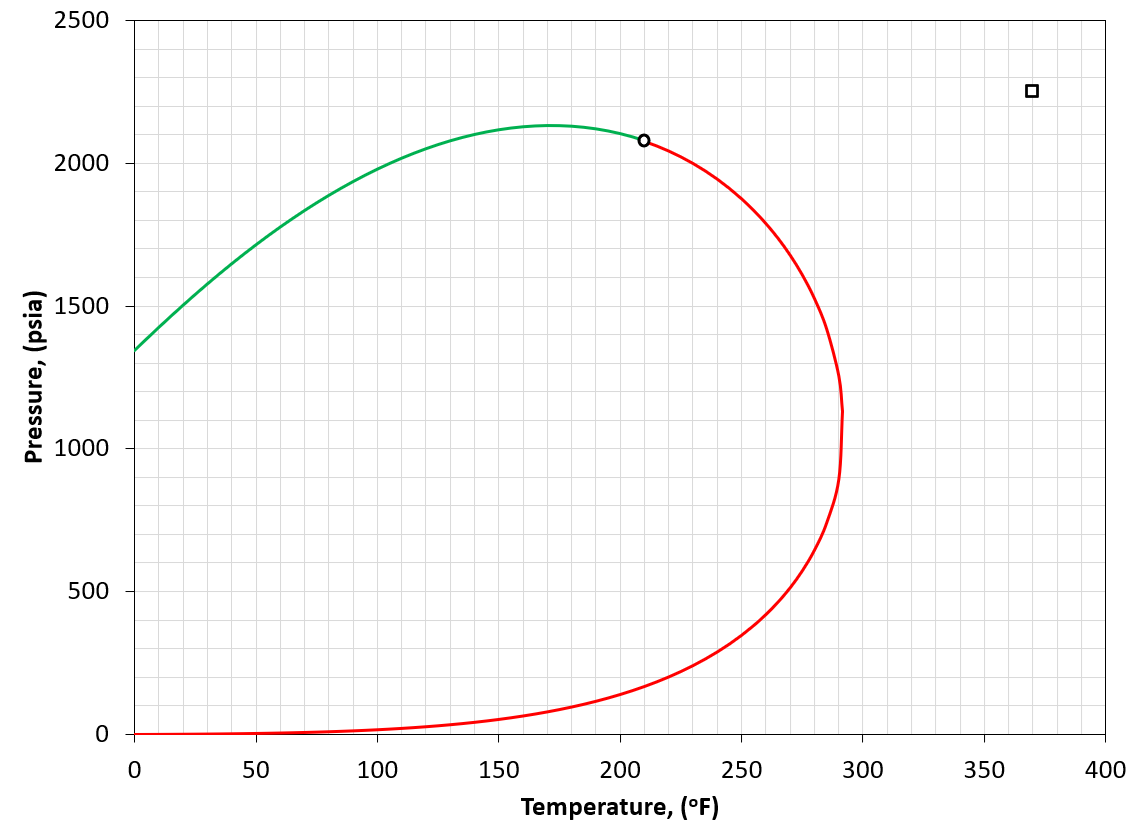Figure 6: An example of a PT phase envelope (bubble point in green and dew point in red) for a dry gas reservoir. The reservoir condition is marked by a black square marker.

A dry gas is defined by having a reservoir temperature much more than the cricondentherm of the reservoir fluid (i.e. $$T_{R}>>T_{cric}$$). An example is given in Figure 6. A dry gas has essentially no produced condensate.

1. C. H. Whitson and M. R. Brulé. Phase behavior. Volume 20. Henry L. Doherty Memorial Fund of AIME, Society of Petroleum Engineers …, 2000.